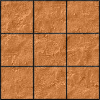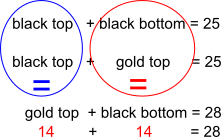#### You may also like### Pyramids

What are the missing numbers in the pyramids?### Paving the Way

A man paved a square courtyard and then decided that it was too small. He took up the tiles, bought 100 more and used them to pave another square courtyard. How many tiles did he use altogether?### Chess

What would be the smallest number of moves needed to move a Knight from a chess set from one corner to the opposite corner of a 99 by 99 square board?

# Black and Gold Storeys

##### Age 11 to 14 Short Challenge Level:

Using reasoningUsing a table$25-a=25-b\therefore a=b$$\therefore a=14$

Using symbols for some of the numbers
Gold storeys in top half: $x$
Black storeys in bottom half: $y$
Given that $x+y = 28$

Gold storeys in the bottom half: $25-x$
Black storey in the bottom half: $y$
$25-x+y=25 \Rightarrow x=y$

So $x+y=28$ means $x$ and $y$ are both $14$, since $x=y$

Using symbols for all of the numbers
$a$ gold storeys in the top half of the building.
$b$ gold storeys in the bottom half of the building.
$c$ black storeys in the top half of the building.
$d$ black storeys in the bottom half of the building.

Then:
$a+b$ = 25   (1)
$c+d$ = 25   (2)
$a+c$ = 25   (3)
$b+d$ = 25   (4)
$a+d$ = 28   (5)

(1) $-$ (4): $a+b-b-d$ = 0 $\Rightarrow a-d$ = 0 $\Rightarrow a$ = $d$

(5) becomes $a+a$ = 28, so $a$ = 14

You can find more short problems, arranged by curriculum topic, in our short problems collection.